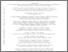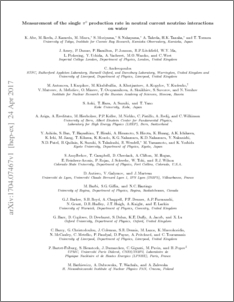# Measurement of the single $π^0$ production rate in neutral current neutrino interactions on water

Abe, K. and Amey, J. and Andreopoulos, C. and Antonova, M. and Aoki, S. and Ariga, A. and Ashida, Y. and Assylbekov, S. and Autiero, D. and Ban, S. and Barbi, M. and Barker, G. J. and Barr, G. and Barry, C. and Bartet-Friburg, P. and Batkiewicz, M. and Berardi, V. and Berkman, S. and Bhadra, S. and Bienstock, S. and Blondel, A. and Bolognesi, S. and Bordoni, S. and Brailsford, D. and Bravar, A. and Bronner, C. and Avanzini, M. Buizza and Calland, R. G. and Campbell, T. and Cao, S. and Castillo, R. and Catanesi, M. G. and Cervera, A. and Chappell, A. and Checchia, C. and Cherdack, D. and Chikuma, N. and Dealtry, T. and Finch, A. J. and Grant, N. and Knox, A. and Kormos, L. L. and Lamont, I. and Lawe, M. and Nowak, J. and O'Keeffe, H. M. and Ratoff, P. N. and Shaw, D. and Short, S. and Southwell, L. (2018) Measurement of the single $π^0$ production rate in neutral current neutrino interactions on water. Physical Review D, 97 (3). ISSN 1550-7998Preview
PDF (1704.07467v1)
1704.07467v1.pdf - Accepted Version
The single $\pi^0$ production rate in neutral current neutrino interactions on water in a neutrino beam with a peak neutrino energy of 0.6 GeV has been measured using the P{\O}D, one of the subdetectors of the T2K near detector. The production rate was measured for data taking periods when the P{\O}D contained water ($2.64\times{}10^{20}$ protons-on-target) and also periods without water ($3.49 \times 10^{20}$ protons-on-target). A measurement of the neutral current single $\pi^0$ production rate on water is made using appropriate subtraction of the production rate with water in from the rate with water out of the target region. The subtraction analysis yields 106 $\pm$ 41 (stat.) $\pm$ 69 (sys.) signal events, which is consistent with the prediction of 157 events from the nominal simulation. The measured to expected ratio is 0.68 $\pm$ 0.26 (stat.) $\pm$ 0.44 (sys.) $\pm$ 0.12 (flux). The nominal simulation uses a flux integrated cross section of $7.63\times{}10^{-39}$ cm${}^2$ per nucleon with an average neutrino interaction energy of 1.3 GeV.View Item